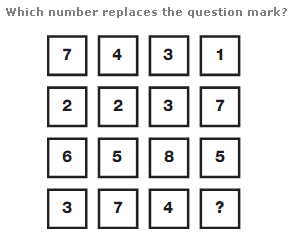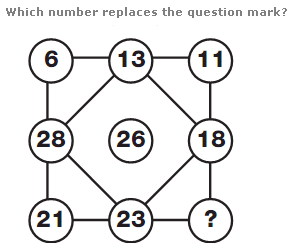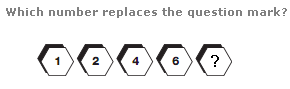# Puzzles - Number puzzles

### Exercise :: Number puzzlesAnswer : 5 Explanation : Working in columns, the sum of the numbers in each column equals 18.Answer : 16 Explanation : Starting on the top left, and moving clockwise in a spiral towards the centre, numbers increase by 7, then decrease by 2. Repeat this pattern all the way around.Answer : 10 Explanation : Working from left to right numbers follow the sequence of Prime Numbers, subtracting 1 each time.